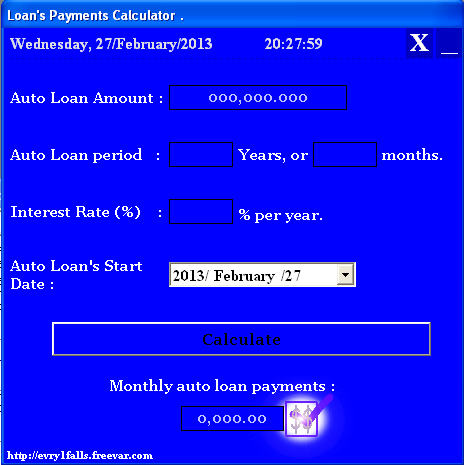Microsoft Visual Basic training - Calculator for monthly payments of a loan

How to calculate the monthly payments of a loan in Visual Basic 6.0Creating a DeskTop application to calculate the monthly payments of a loan

Introduction

What is Monthly Payments of a loan?

Buying cars, new houses, having a pool or even a garden is every man's dream, unless you were born so rich to afford this, or you can go and get yourself a loan, this is simply what loan is. It's an amount of money that you get from a bank in order to be able to buy yourself a life (hahahaha) if you don't have one, but the catch is that you will have to pay later and you also need to be financially secured, so the bank could trust to give you such loan, anyway this is not our point.

The bank where you will get your loan from is going to calculate some payback amounts called (monthly payment) which are the interest of the loan you have just ordered, these monthly payments is our target.

- In order to calculate the monthy payment of a loan you need to know 3 elements related to this loan :

1. How much is the loan amount ?
Is how much the bank will give to you as a loan.
2. The Interest Rate.
On what basis these monthly payments are going to be calculated, or it's some percentages % to determine the monthly payments per month
3. The period of the loan
Is how long untill the loan is to be repaid to the bank, or it is the number of months that you will pay your monthly payments (all the monthly payments)

The Interest Rate is given by the bank, each loan system has its own Interest Rate. Interest Rates vary from bank to another and from loaner company to another, it depends on the company's or bank's willing to keep the money in your hands before they collect it + Interest .

Calculations

How does it work?

There are several ways to calculate the monthly payments, the accountant of the bank has its own way, so as the loaner comapny's accountant, so as the Excel user and the programmers of course have their own way.

It is a calculator, but not just any calculator, it will be made to do certain calculations resricted to certain rules, these rules are the (3 elements) we talked about above. You will need to get the Monthly Payments as an output and you will have (Loan's amount - Interest Rate - Loan's period in years or months) as inputs .

Math (Manually) calculations

Monthly payments of a \$12,000 for 1 year by Interest Rate of 10% is calculated like this :

P = (Pv*R) / [1 - (1 + R)^(-n)]
where
Pv = Present Value (beginning value or amount of loan)
*APR = Annual Percentage Rate (one year time period)
R = Periodic Interest Rate = APR/ # of interest periods per year
P = Monthly Payment
n = # of interest periods for overall time period (i.e., interest periods per year * number of years)

Using Microsoft Excel built-In function

Even simple : Using Microsoft Excel built-In function [PMT], Syntax :

= pmt(10%/12 , 12, 12000)
=1054.99\$

Where
- (10% / 12) is the interest equation.
- 12 is the period (how many times we will pay the bank each year) for one year.
- 12000\$ the amount of the loan

- We will use the manual calculations, because Microsoft Excel built-In function uses the same manual calculations implicitly to create such handy formula for its users. As a programmer you need to analyze yourself and come up with your own formulas. Here is what I come up with.

Project Design

What is the project design ?Our Visual Basic 6.0 project example is about creating a Calculator to calculate the monthly payments of a loan, and I keep saying that I don't know why.

The project design is just a form and a button [Calculate] and a Function to be able to move the form from any place on it without using the title bar and [Date/Time] function in the head of the form, and finally our inputs :

- The interest Rate
- The amount of the loan
- The period [Months] and [Years]

When you click [Calculate] you get the monthly payments of the amount you entered in the [TextBox] amount according to the other inputs.

Source Code

Source Code Highlights

This code enables you to move the form from any place on it using the mouse cursor :

This code will enable you to format the Date/Time label :

Downloan the source code from a direct link from Mediafire.com